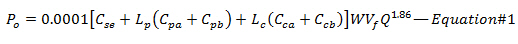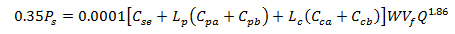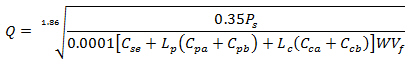# Determine Optimum Drilling Flow Rate for Basic System – Drilling Hydraulics

From the previous post (total pressure loss excluding the bit), we know the equation (shown below)We can solve the equation to get the flow rate (Q) that match with optimization criteria (Maximum Hydraulic Horsepower or Maximum Impact Force)

Maximum Hydraulic Horsepower will be happened when the pressure drop across the bit is equal to 65% of total pressure loss; therefore, 35% of total pressure loss (Ps) occurs through the drill stem (Po). The equation will be like this Po = 0.35 x Ps. By substituting Po with 0.35Ps into the Equation#1, you can solve for the optimum flow rate which will give you the maximum hydraulic horsepower.Solve for QMaximum Impact Force can be obtained when 48% of total pressure loss is at the bit so 52% of total pressure loss (Ps) occurs through the drill stem (Po). Substituting 52% of Ps into the Equation#1 will allow you to get the optimum flow rate at maximum impact force.Solve for QWhere:

Ps = Surface Pressure

Cse =Surface Coefficient

Lp = Length of Pipe

Cpa = Coefficient for the annulus of pipe

Cpb = Coefficient through inside of pipe and tool joint

Lc = Length of Drill Collar

Cca = Coefficient for the annulus of drill collar

Ccb = Coefficient through inside of drill collar and tool joint

W = mud weight in ppg

Vf = Viscosity Correction Factor

Note: Vf can be determined by this equation: Vf = (PV÷W)0.14

Reference:Drilling Hydraulic Books

Share the joyWorking in the oil field and loving to share knowledge.

### 2 Responses to Determine Optimum Drilling Flow Rate for Basic System – Drilling Hydraulics

1.Johan says:

How do you determine the coefficient of surface equipment?

•DrillingFormulas.Com says:

Johan,